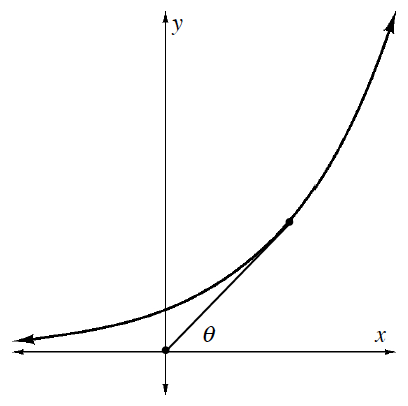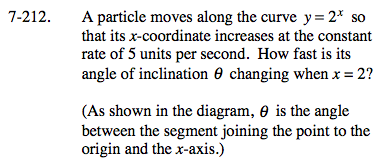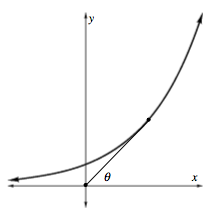### Home > CALC > Chapter 7 > Lesson 7.4.4 > Problem7-212

7-212.
1. A particle moves along the curve y = 2x so that its x-coordinate increases at the constant rate of 5 units per second. How fast is its angle of inclination θ changing when x = 2?

2. (As shown in the diagram, θ is the angle between the segment joining the point to the origin and the x-axis.) Homework Help ✎$\text{tan}\theta =\frac{\text{opposite side}}{\text{adjacent side}}$

What are the opposite and adjacent sides here?To obtain θ', you will have to use implicit differentiation in terms of t, since the x-coordinate and θ are changing over time.

$2\text{ln}2-1\approx 0.386$